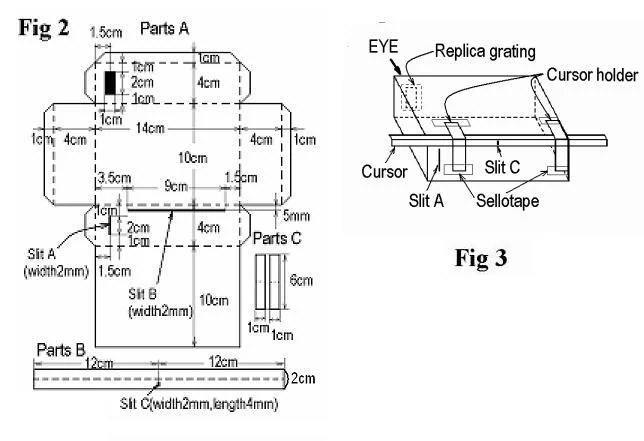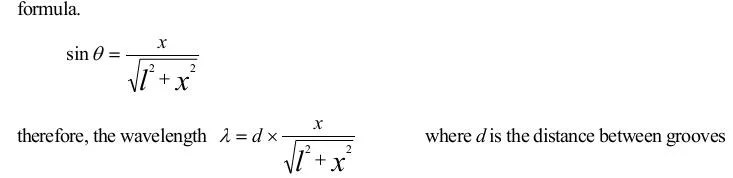## Make a Handmade Spectroscope Measure a Light Wavelength!

Concept: Light is a kind of wave How Do You Measure a Light Wavelength?

### Introduction:

As you know, light is a kind of electromagnetic waves and its wavelength is 400 nm(Violet)～800nm(Red). T.Young measured the light wavelength for the first time
using interference of light by double slits. But, we can measure the light wavelength more easily using a diffraction grating. So let’s make a handmade spectroscope using a piece of replica grating in order to measure the light wavelength.

### Materials:

(a) cardboard (size 25 cm×30 cm) (b) a piece of replica grating (1000grooves/mm, size 2.5 cm×2.5 cm)  Fig.1 Finished product (c) a black permanent marker (d) a cutter/scissors   (e) wood glue    (f) sello tape

### Construction:

1. Draw the figures of parts A,B and C on the cardboard as shown in Fig.2.
2. Cut out parts A, B,C and paint the back side of them black using a black permanent marker.
3. Make two slits and a rectangular hole on parts A. Make a slit C on parts B (cursor).
4. Fold parts A along the dotted lines shown in Fig.2. Using wood glue, make a box whose inside is painted black  as shown in Fig.3
5. Attach the replica grating film on the rectangular hole of the box from outside using sellotape as shown in Fig.3.
6. Using sellotape, attach parts C (cursor holder) to the box as shown in Fig.3.
7. Fold parts B(cursor) along the dotted centre line and insert it to the cursor holders.
8. Direct slit A to a light source with youreye in contact with the replica grating and you can see a spectrum. Measure the light wavelength by moving the cursor.( How to measure is written in the following page )### Science:

Light is a kind of wave. Diffraction and interference are the nature of waves. Because of these two natures of light, we can see a spectrum using a diffraction grating. As shown in Fig.4, when parallel light rays come into a diffraction grating perpendicularly, they are diffracted by the grooves of a diffraction grating. Diffractive rays whose diffractive angle isθstrengthen each other by interference if theysatisfy the following formula: dsinθ=mλwhere d=distance between grooves,λ=wavelength,m=integer When these diffractive rays come into our eyes, they appear as if theyare coming from Q. If the diffractive light rays which satisfy the above formula are green, wecan see green in the direction of Q.Next, I would like to describe how to measure the light wavelength using this apparatus. When you contact the replica grating of the apparatus to your eye and direct slit A to the light source, you see the spectrum of the first order (m=1) as shown in Fig.5. If you want to measure the wavelength of green light, you should move the cursor so that the light from the hole of the cursor is just vertically above the green belt of the spectrum. Then, measure x, the distance between slit A and the hole of the cursor. If the depth of the apparatus isl, the value of sinθis given by the following formula.If the replica grating has 1000 grooves per millimeter, the value of dis 1/1000 mm. Thus we can get the value of the wavelength using this apparatus.

### Things to do:

Using this apparatus, measure the light wavelengths of green line and violet line in the spectrum of
a fluorescent light.
(There are many mercury atoms in a fluorescent bulb. The spectrum of light emitted from atoms
consists of many lines and it is called “Line Spectrum”)